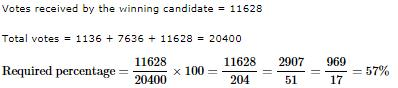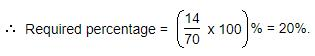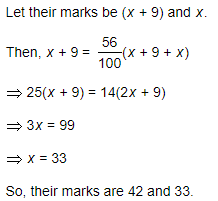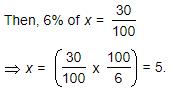Courses

# Practice Test: Percentages- 2

## 10 Questions MCQ Test SSC CGL Tier 2 - Study Material, Online Tests, Previous Year | Practice Test: Percentages- 2

Description
This mock test of Practice Test: Percentages- 2 for Banking Exams helps you for every Banking Exams entrance exam. This contains 10 Multiple Choice Questions for Banking Exams Practice Test: Percentages- 2 (mcq) to study with solutions a complete question bank. The solved questions answers in this Practice Test: Percentages- 2 quiz give you a good mix of easy questions and tough questions. Banking Exams students definitely take this Practice Test: Percentages- 2 exercise for a better result in the exam. You can find other Practice Test: Percentages- 2 extra questions, long questions & short questions for Banking Exams on EduRev as well by searching above.
QUESTION: 1

Solution:

x%ofy=x/100×y
=y/100×x
=y%ofx
∴A=B

QUESTION: 2

### Three candidates contested an election and received 1136, 7636 and 11628 votes respectively. What percentage of the total votes did the winning candidate get?

Solution:QUESTION: 3

### What percentage of numbers from 1 to 70 have 1 or 9 in the unit's digit?

Solution:

Clearly, the numbers which have 1 or 9 in the unit's digit, have squares that end in the digit 1. Such numbers from 1 to 70 are 1, 9, 11, 19, 21, 29, 31, 39, 41, 49, 51, 59, 61, 69.

Number of such number =14QUESTION: 4

Two tailors X and Y are paid a total of Rs. 550 per week by their employer. If X is paid 120 percent of the sum paid to Y, how much is Y paid per week?

Solution:

X+ y=Rs. 550
x = 120% of y
120% * y+ y =550
120/100*y+y = 550
6/5y+y =550
6y+5y/5 = 550
11/5y = 550
11y = 550*5
y = 250

QUESTION: 5

Two numbers A and B are such that the sum of 5% of A and 4% of B is two-third of the sum of 6% of A and 8% of B. Find the ratio of A : B.

Solution:

5100A+4100B=23[6A100+8B100]

5A+4B=2/3(6A+8B)

15A+12B=12A+16B

3A=4B

A/B=4/3⇒

A : B = 4 : 3

QUESTION: 6

In an election between two candidates, one got 55% of the total valid votes, 20% of the votes were invalid. If the total number of votes was 7500, the number of valid votes that the other candidate got, was:

Solution:

Total Valid votes are = 80% of 7500= 6000
If one has 55% vote then the other has left with 45% votes
Hence, 45% of 6000= 2700

QUESTION: 7

Q. Two students appeared at an examination. One of them secured 9 marks more than the other and his marks was 56% of the sum of their marks. The marks obtained by them are:

Solution:QUESTION: 8

Gauri went to the stationers and bought things worth Rs. 25, out of which 30 paise went on sales tax on taxable purchases. If the tax rate was 6%, then what was the cost of the tax free items?

Solution:

Let the amout taxable purchases be Rs. x.∴ Cost of tax free items = Rs. [25-(5+0.30)] = Rs. 19.70

QUESTION: 9

A fruit seller had some apples. He sells 40% apples and still has 420 apples. Originally, he had:

Solution:

x-x(40/100)=420
x-40x/100=420
or, 60x/100=420
x=42000/60
x= 700

QUESTION: 10

If 20% of a = b, then b% of 20 is the same as:

Solution:

20% of a = b  => (20/100)a = b

b% of 20 =(b/100) x 20 = (20a/100) x (1/100) x (20) = 4a/100 = 4% of a.